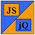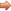# JavaScript & jQuery Tutorials

JS HomeJS IntermediateConditional Statements

## Conditional Statements

There comes a time within a program, where we need to do something based on operand comparison, for this we use conditional statements. In this lesson we look at the `if....else` construct for basic conditional handling. All premutations of the `if....else` construct just use one of the comparison operators available in Javascript to test one operand against another.

All the comparison operators are discussed in detail in JavaScript Reference - Comparison Operators so we won't go into them here.

There are various forms of the `if....else` construct which we will cover as we go through this lesson.

## Simple `if` Statement

The basic `if` statement compares the operands and returns boolean `true` or `false`.

If the boolean is `true` the statement(s) are executed.

``````

var aVariable = 1, bVariable = 1;

// Compare some operands.
if (aVariable == bVariable) {
}

``````

Press the button below to action the above code:

## Single `if....else` Construct

The single `if....else` construct compares the operands and returns boolean `true` or `false`.

If the boolean is `true` the statement(s) following the `if` are executed.

If the boolean is `false` the statement(s) following the `else` are executed.

``````

var aVariable = 1, bVariable = 2;

// Compare some operands.
if (aVariable == bVariable) {
} else {
}

``````

Press the button below to action the above code:

## Multiple `if` Construct

The multiple `if` construct compares the operands and returns boolean `true` or `false` for each statement.

If the boolean is `true` the statement(s) following the `if` or `else....if` are executed.

If the boolean returned is `false` the next `else....if` statement(s) is checked and so on.

If none of the `else....if` statements returns `true` the final `else` statement(s) will be false and so any statement(s) after this will be executed.

``````

var aVariable = 4, bVariable = 2, cVariable = 3, dVariable = 4;

// Compare some operands.
if (aVariable == bVariable) {
} else if (aVariable == cVariable) {
} else if (aVariable == dVariable) {
} else {
}

``````

Press the button below to action the above code:

## Lesson 3 Complete

In this lesson we looked at the `if....else` construct and the various ways we can make use of it.

## Related Tutorials

In the next lesson we take our first look at JS loops with the `while` and `do....while` loops.